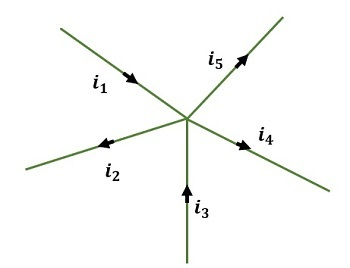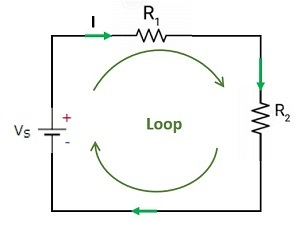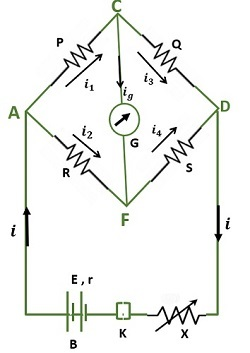# Generalization of Kirchhoff's Laws

## Introduction

Kirchhoff’s law is used to study the electrical energy used in a circuit. The movement of the charged particles is responsible for electric energy. Energy obtained from the kinetic energy or potential energy of the charged particles is the electric energy. Charged particles are atoms, molecules, or ions that have a charge on them.

There is a force of attraction or repulsion between the charged particles which depends on the coulomb’s law. Electricity flow from one point to another point through a closed path called a circuit. Electric current passes through this closed path. We use many electronic components such as a transistor, resistor, capacitors, etc. Many electronic devices like computers, DVD players etc. are composed of these electronic.

An electrical circuit that is represented graphically is called a circuit diagram. The main application of circuit diagrams is designing, constructing, and maintaining electrical and electronic circuits.

## What are Kirchhoff’s Laws?

Gustav Robert Kirchoff was a German physicist who developed circuit laws. He conducted research in many fields of physics such as electric circuits, spectroscopy, and black body radiation.

## Kirchhoff’s First Law

Kirchhoff’s first law is about the current that flows through the circuit. It is also denoted as Kirchhoff’s current law (KCL). According to this law, the total amount of current or the charges that enter the junction or a node is the same as the amount of current or the charges that exit the junction or a node when there is no loss of charge at the node. It can also be stated as the addition of the current that flows in and out of the circuit is equal to zero. It works on the principle of the law of conservation of charges. Thus by the first law of Kirchhoff,

$$\mathrm{the\:sum\:of\:current\:entering\:the \:circuit=sum\:of\:current\:leaving\:the\:circuit}$$

Let us apply Kirchhoff's first law in the following circuit then we get,

$$\mathrm{i_1+i_3+i_4=i_2+i_5}$$

By taking the current entering the circuit as positive and leaving the circuit as negative it is also expressed in another form such as

$$\mathrm{i_1-i_2+i_3+i_4-i_5=0}$$Fig:1 Junction law

Kirchhoff’s law is applies to distributed networks. It does not depend upon the nature of the networks such as active, passive, linear, or nonlinear. Current always flows in the path which has low resistance.

## Kirchhoff’s second Law

Kirchhoff’s second law states the voltage drop across the circuit. It is also denoted as Kirchhoff’s voltage law. It is the consequence of both conservations of charges and conservation of energy. The addition of voltage across a closed loop of the circuit in a specific direction is zero. By Ohm’s law voltage across the component is $\mathrm{V=IR}$

Let us apply Kirchhoff’s second law in the following closed loop we get,Fig:2 Voltage law

$$\mathrm{V_s=IR_1+IR_2}$$

KVL is used for all circuits irrespective of the nature of the circuit. It is used in the principle of conservation of charge and energy. In a parallel path, the voltage is constant and in the series path, it is divided.

## Generalization of Laws

Initiation of general truth based on observation or experiments that are made between any two phenomena is called generalization of laws. By doing multiple experiments the laws are generalized.

## Uses

Kirchhoff’s is used in many areas. If the current passes through the circuit there is a voltage drop across the different circuit areas. Kirchhoff’s law is used to find the amount of current that flows through the circuit and the voltage drop across the circuits. It gives the direction of the current that flows through the circuit.

Kirchoff’s law describes the distribution of current in circuits containing many components. It has a way to find the voltage in complicated DC circuits. By this law, one can find the internal resistance of the circuit. One of the best applications of Kirchhoff’s first law is the Wheatstone bridge.

## Application

One of the applications of Kirchhoff’s law is Wheatstone’s bridge to find the unknown resistance. it uses both laws. Let us consider four resistance P, Q, R, and S that are connected in a closed loop with the battery and galvanometer in the following manner. Let the current that passes through the resistances P, Q, R, and S are $\mathrm{i_1,i_3,i_2}$,and $\mathrm{i_4}$. The current passing through the galvanometer is ig. Here Kirchhoff's law is applied to junctions C and F.Fig:3 Wheatstone bridge

By applying this at the junction C we get,

$$\mathrm{i_1-i_3-i_g=0}$$

$$\mathrm{i_1=i_3+i_g………………………………(1)}$$

At the junction F, we get,

$$\mathrm{i_2+i_g-i_4=0}$$

$$\mathrm{i_4=i_2+i_g………………………………..(2)}$$

At equilibrium condition ig =0. Then (1) and (2) will become

$$\mathrm{i_1=i_3}$$

$$\mathrm{i_4=i_2}$$

Thus Kirchhoff's first law is applied in a part of Wheatstone’s bridge.

Applying the second law in the loops ACFA and CDFC we get,

$$\mathrm{i_1 P+i_g G-i_2 R=0……………………………(3)}$$

$$\mathrm{i_3 Q-i_4 S-i_g G=0 ………………………(4)}$$

At equilibrium condition ig =0. Then (3) and (4) will become

$$\mathrm{i_1 P\:-\:i_2 R\:=\:0}$$

$$\mathrm{i_1 P=i_2 R………………………..(5)}$$

$$\mathrm{i_3 Q-i_4 S=0}$$

$$\mathrm{i_3 Q=i_4 S……………………………..(6)}$$

Divide equation (5) by (6)

$$\mathrm{\frac{i_1 P}{i_3 Q}=\frac{i_2 R}{i_4 S}}$$

Here $\mathrm{i_1=i_3}$ and $\mathrm{i_4=i_2}$ So

$$\mathrm{\frac{i_1 P}{i_1 Q}=\frac{i_2 R}{i_2 S}}$$

$$\mathrm{\frac{P}{Q}=\frac{R}{S}}$$

By this formula, the unknown resistance in the circuit can be calculated.

## Limitations

Kirchhoff’s laws are applicable only for high-frequency AC circuits because of the induced emf due to the magnetic field. Kirchhoff’s current law is applicable for the circuit where the current through the circuit is constant. If there is an assumption that the magnetic field is not changing in a closed circuit, then only the KVL is applicable. It operates on the condition that the electric field in the circuit is neglected.

## Conclusion

In this tutorial, Kirchhoff's laws and the generalization of the law were discussed. The uses of Kirchhoff’s laws and the limitations of Kirchhoff’s laws are also discussed in detail.

## FAQs

Q1. What are the characteristics of an electric circuit?

Ans. There must be a return path in the electric circuit as it is a closed loop. In every electric circuit, there should be at least one power source. That is it should contain at least one active element. Electric circuits contain both active and passive elements connected in series or parallel. In an electric circuit, the electron flows from the negative terminal to the positive terminal.

Q2. What is the law of conservation of charge?

Ans. It states that if the circuit is isolated then the total charges in the circuit do not change and it remains constant. In other words, it can also be illustrated as the charges cannot be created or destroyed.

Q3. What is conservation of energy?

Ans. By the conservation of energy law, one form of energy can be transformed into another form and the energy cannot be destroyed or formed. In other ways, it can also be stated that if the system is isolated then the total energy of the system does not change and it remains constant.

Q4. State Ohm’s law

Ans. It gives the relation between the current through the circuit and the voltage across the circuit. It gives the proportionality between the current and the voltage when all physical conditions including temperature are constant. the Ohm’s law equation is

$$\mathrm{V=IR}$$

R is the proportionality constant and it is the resistance of the circuit for the current flow.

Q5. What are the applications of Ohm’s law?

Ans. It is used to divert the current in the DC ammeter and DC shunt. The current, voltage, and resistance of the circuit are calculated using Ohm’s law. Preferred voltage drop is maintained using Ohm’s law.

Updated on: 06-Apr-2023

183 Views